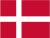### Dish Cloth 38

Cast on 60 stitches and knit 4 rows, and then pattern. Remember to start and end all rows with 2 k.
When the cloth has the desired length - knit 4 rows and bind off.

Pattern:
1.row: 1 k, 6 p, 1 k
2.row: 3 k, 2 p, 3 k
3.row: 2 p, 4 k, 2 p
4.row: as row 1
5.row: 1 p, 6 k, 1 p
6.row: as row 1
7.row: as row 3
8.row: as row 2
9.row: as row 1
10.row: as row 3
11.row: as row 2
12.row: 3 p, 2 k, 3 p
13.row: as row 2
14.row: as row 3

 X X X X 14 X X 13 X X 12 X X 11 X X X X 10 X X X X X X 9 X X X X X X 8 X X X X 7 X X 6 X X 5 X X 4 X X X X 3 X X X X X X 2 X X X X X X 1
You have to remember that all uneven rows start in the right side of the diagram and X=purl and ▢= knit. All equal rows start in the left side of the diagram and X=knit and ▢=purl.Danish